An often-misunderstood concept in game theory is the zero-sum game. Let’s take a look at what it is.

In a zero-sum game, one participant’s gains are offset by losses from the other players. In other words, if you add up all of the winnings and all of the losses, they will equal zero.

This isn’t true in all games. For example, in the Prisoner’s Dilemma examples we saw previously, both players can lose or both players can win.

To illustrate a zero-sum game, let’s think of poker. Four players start with \$25 each, meaning a total of \$100.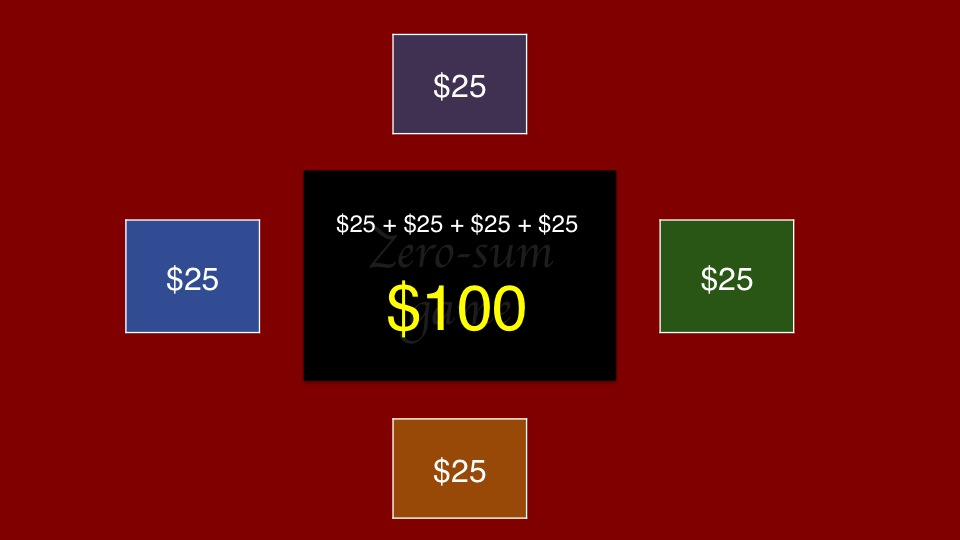Orange starts the betting at \$2. Blue calls. Purple folds. Green calls. There is now \$6 in the pot: each of the three players in the hand has contributed \$2, leaving each with \$23.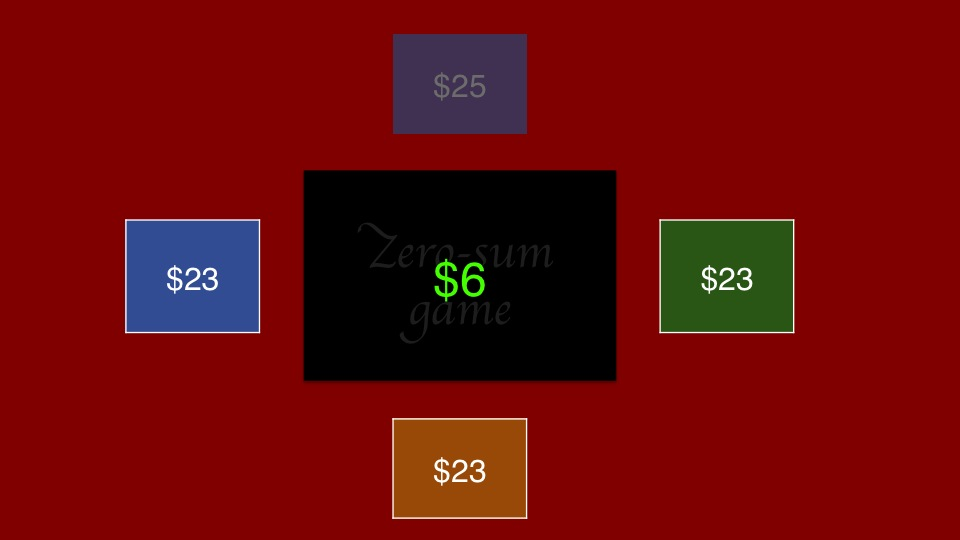Orange goes first again, and forces the remaining two players to fold with a bet of \$10. He adds the \$16 on the table to the \$13 still in front of him, giving him a total of \$29.

If we add each player’s total, we see there’s still \$100 on the table.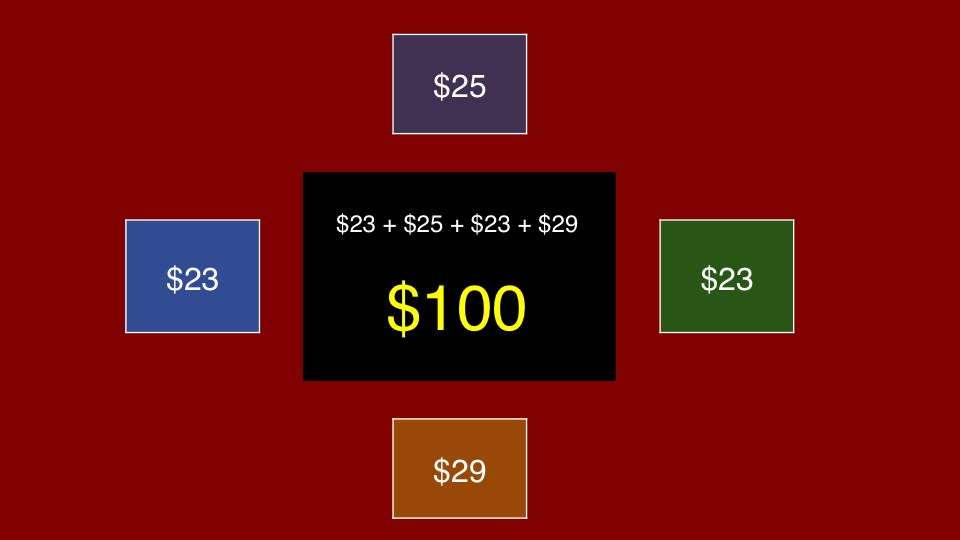The term “zero-sum” comes from looking at the difference from what each player started with. Blue has lost \$2, purple is even, Green has lost \$2, and Orange has made \$4. If we add those differences together we get zero. The total gains of \$4 are offset by the total losses: two players having lost \$2 each.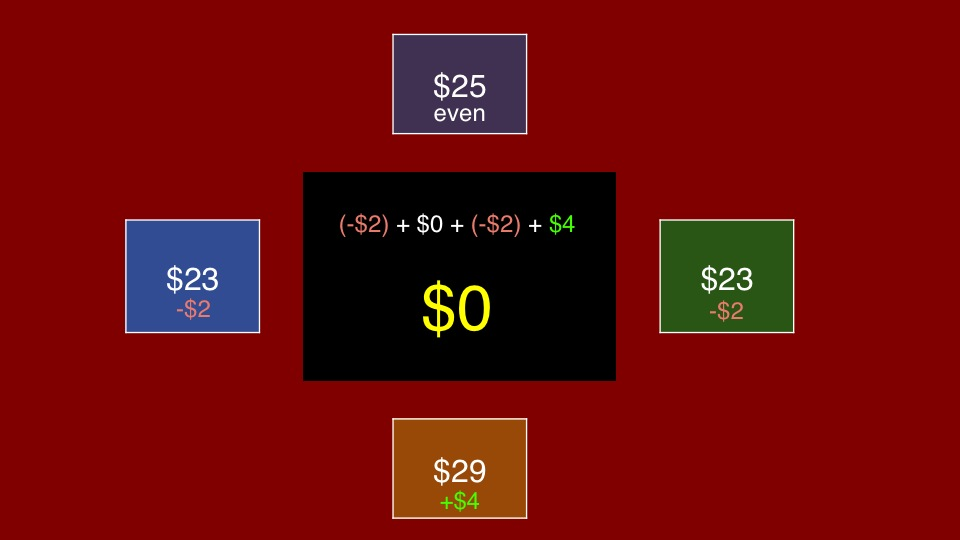When analyzing a two-player Jeopardy! situation, we’ll assume a win is worth plus one, and a loss is worth negative one. We’ll also assume for the sake of simplicity that no ties are allowed.

Here is the situation we looked at in the Nash equilibrium guide. The leader has 20,000 and the trailer has 14,000. Each has two options from which to choose: a large wager, and a small wager.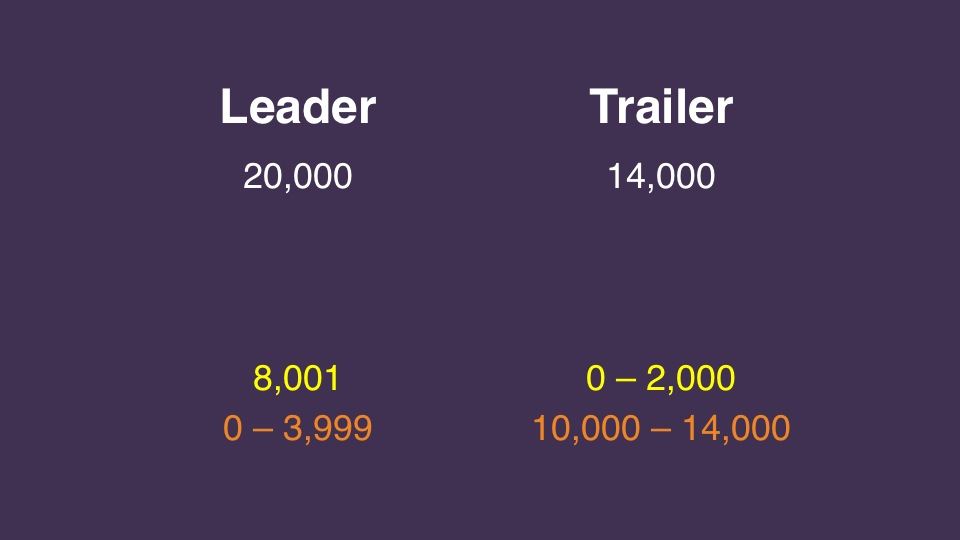We will work through the payoff matrix for this situation. The leader, represented in the rows, can wager either small – zero – or large – 8,001. The trailer, represented in the columns, can wager either small – zero – or large – 14,000. (Any wager in the same range as our chosen values will have the same outcomes.)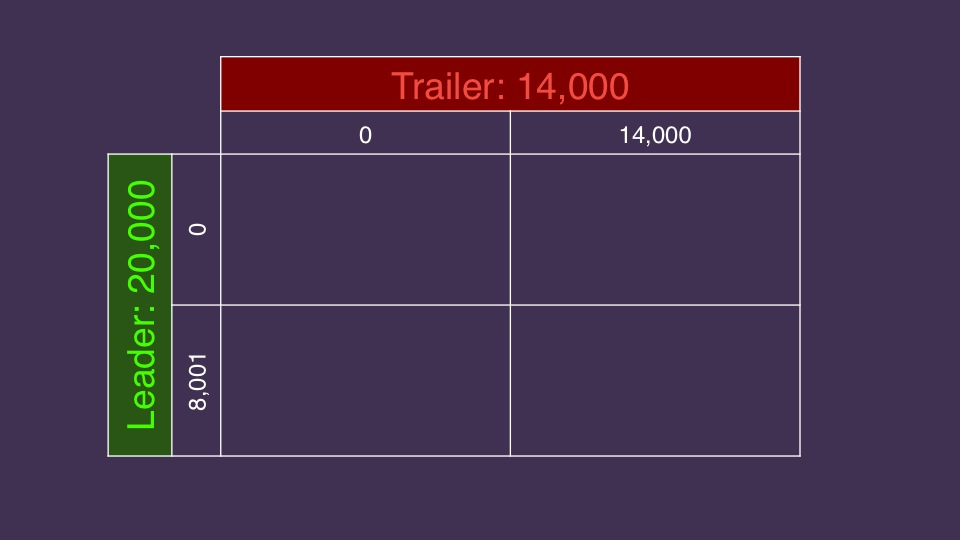Each player will be either right or wrong, and we’ll assume a 50% probability of each (like flipping a coin). That means there are four combinations of how our two players can respond: both are right, the leader is right and the trailer is wrong, the leader is wrong in the trailer is right, and both players are wrong.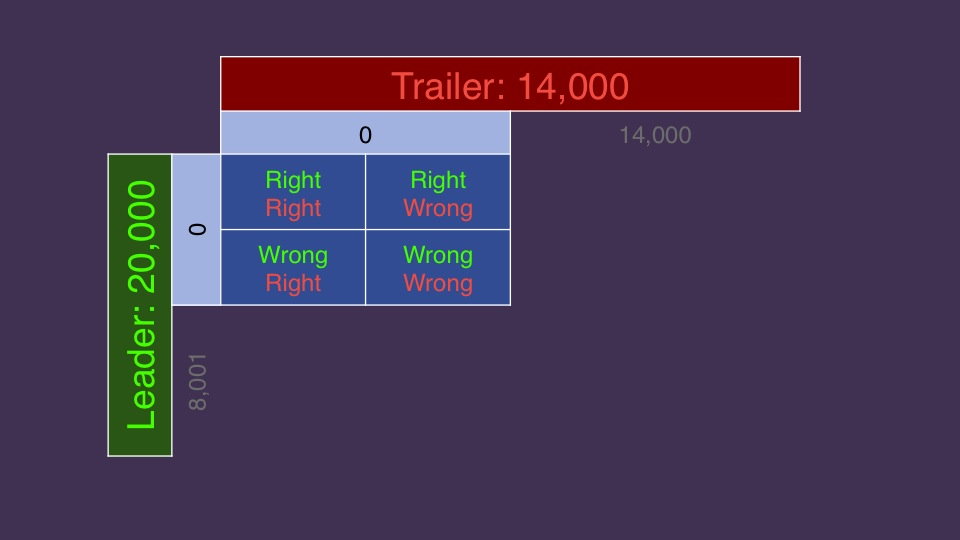When both players wager zero, no matter how each responds, the leader will have 20,000 and the trailer will have 14,000. That means the leader will win in every case.We replace each score combination with the payoff matrix notation: +1 for the winner, -1 for the loser. We do this for all possible outcomes, giving us 16 possibilities, in which the leader wins 11 times.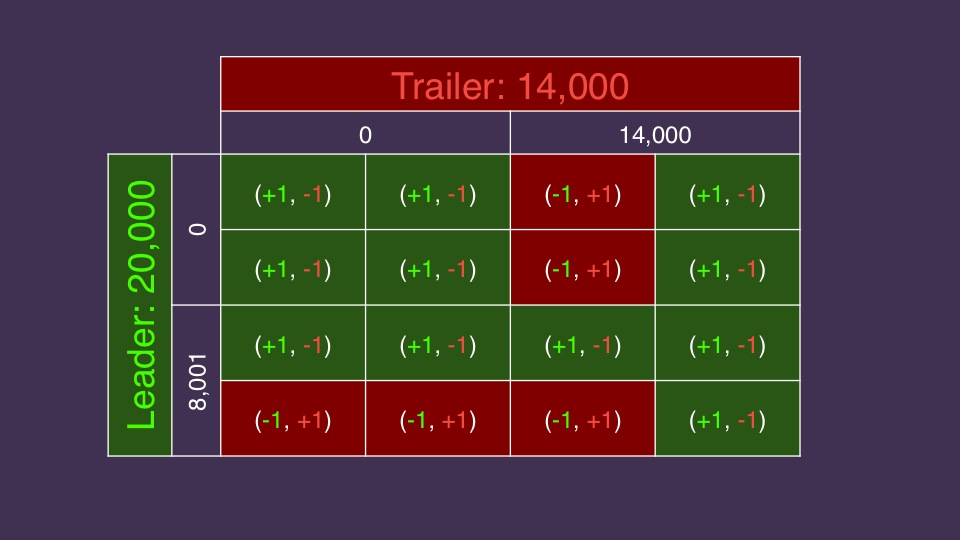We look at the intersection for each individual decision, mindful that each combination is equally likely. For example, where both players wager small, the leader wins every time, so his total score is +4; the trailer’s score is -4.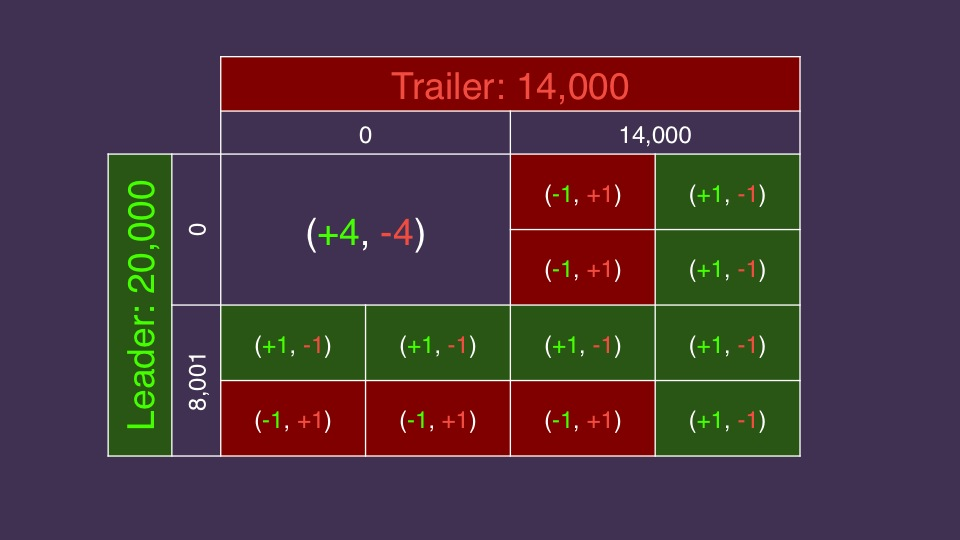We can see that this will not have a Nash equilibrium that’s immediately obvious to us. If we start at the bottom left corner, we’ll see the leader can do better if he wagers zero if he thinks the trailer will wager zero. And as we saw previously, we will keep circling around the matrix with no end in sight.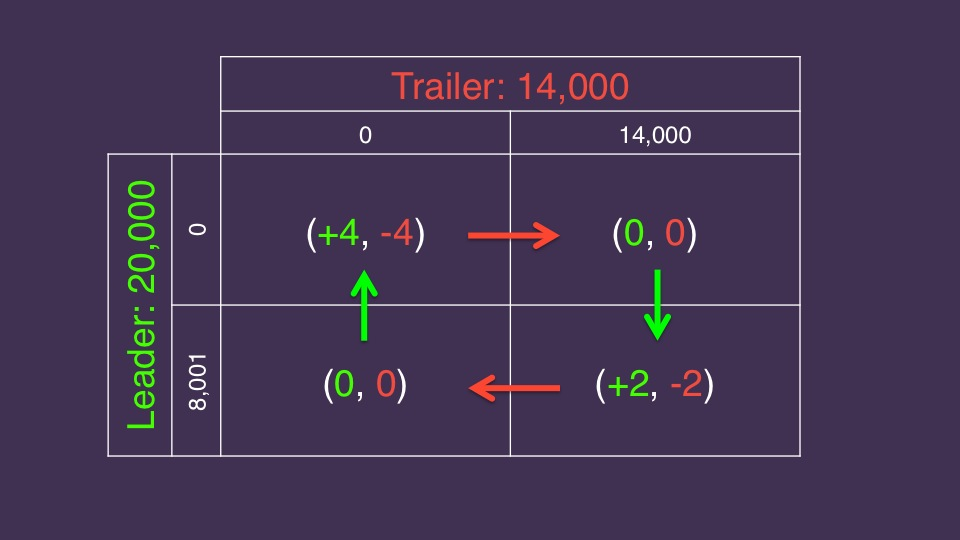But it does have one – and it involves a bit of randomness. We’ll look at that next time!